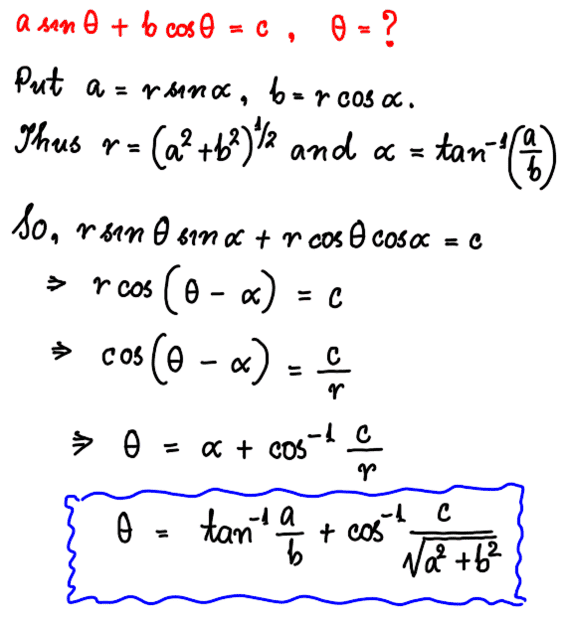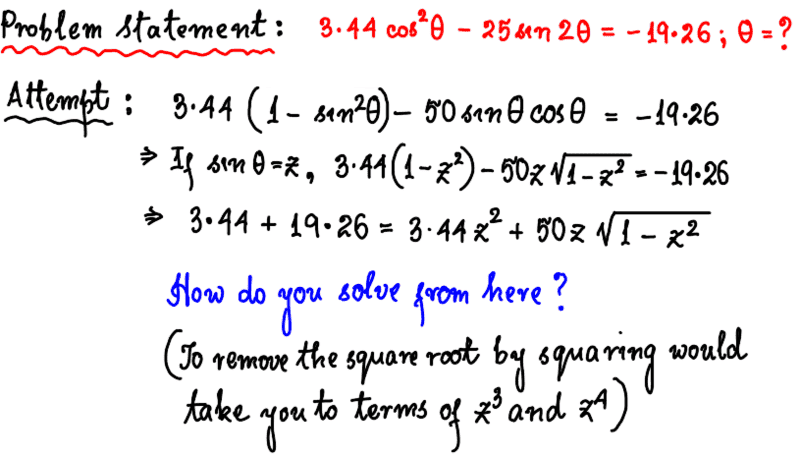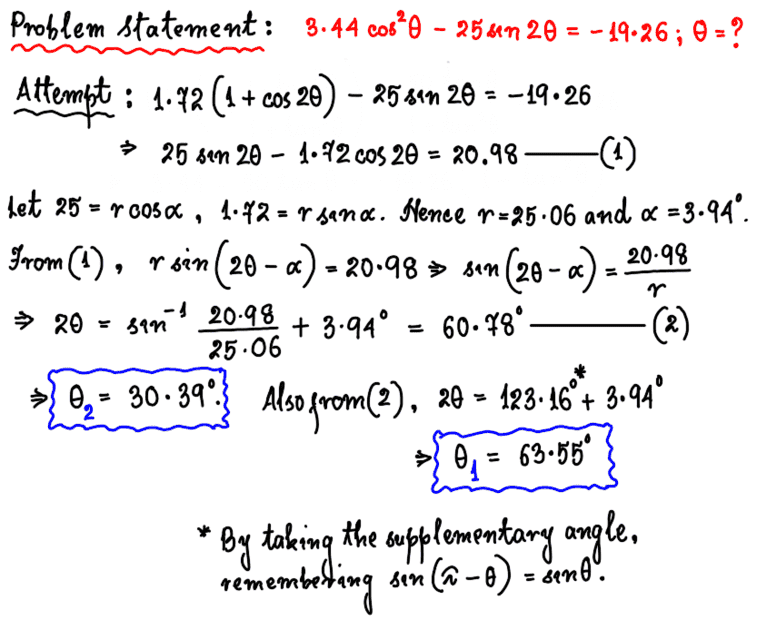# Help Solving a Trigonometric Equation

• vibha_ganji
In summary, the two principal value answers to the equation are ##\boxed{\theta_1 = 62.7^{\circ}\; , \; \theta_2 = 31^{\circ}}##.f

#### vibha_ganji

Homework Statement
3.44(cos^2 (theta)) - 25 sin(2 * theta) = -19.62
Relevant Equations
cos ^2 theta = cos theta times cos theta
sin (2* theta) = sin theta times cos theta times 2
I’m stuck on how to begin. I’ve tried to factor out sin theta from both of the terms on the left hand side but that led to nowhere. Could I have a hint on how to continue? Than you!

•Delta2
Try substituting ## \sin^2{\theta}=1-\cos^2{\theta} ##, and the rest is algebra.
My mistake, I misread the problem. Try ## \cos^2{\theta}=(\cos(2 \theta)+1)/2 ##.
## a \cos(2 \theta)+b \sin(2 \theta) ## then simplifies with an algebraic "trick".

•Delta2
One additional hint on the algebraic trick, because it is not obvious unless you have seen it before:
##a \cos{\theta}+b \sin{\theta}=\sqrt{a^2+b^2} \cos(\theta-\phi) ## where ## \phi=\arctan(b/a) ##.
Be sure and prove to yourself the formula I just gave you.

•Delta2
@vibha_ganji Were you successful at solving it? We welcome your feedback. :)

•SammyS
One additional hint on the algebraic trick, because it is not obvious unless you have seen it before:
##a \cos{\theta}+b \sin{\theta}=\sqrt{a^2+b^2} \cos(\theta-\phi) ## where ## \phi=\arctan(b/a) ##.
Be sure and prove to yourself the formula I just gave you.
I have managed to do the original problem (See original post #1) using a slightly different method than the one you (@Charles Link) mentioned. I will wait and see @vibha_ganji 's effort first before giving my own. However, in your post #3 above, you mentioned a different point that got me thinking about it as a separate problem in its own right. Let me type it below.

Problem : If ##\pmb{a\cos\theta+b\sin\theta=c}##, what is ##\pmb{\theta=?}##.

Attempt : I copy and paste my solution below using a well known "trick" I learned in high school where you express both ##a## and ##b## in terms of sine's and cosine's of the same angle (say ##\alpha##) times a variable (say ##r##).•@vibha_ganji : While I won't tell you how, I will give you the answers to your problem. I would also advise you to learn how to type mathematics on here (using ##\mathbf{\LaTeX})## so as to make your questions and solutions look more readable.

Your problem statement : Solve the trigonometric equation ##3.44\cos^2\theta-25\sin 2\theta=-19.62##.

Solution : ##\text{I leave this for you.}##

Answers : I find there are two answers : ##\boxed{\theta_1 = 62.7^{\circ}\; , \; \theta_2 = 31^{\circ}}##. Both values of ##\theta## plugged into the left hand side of the given equation yield the value on the right hand side (##-19.62##).

•I find there are two answers
I think you can also add any multiple of ## 180^{\circ} ## to either of those two answers and it will also be a solution.

and one alternative solution would be to write ## \sin(2 \theta)=2 \sin{\theta} \cos{\theta} ##, and use ## \sin{\theta}=\pm \sqrt{1-\cos^2{\theta}} ##, but that gets rather clumsy with the algebra.

Edit: In any case, I solved it this way also, and got the same answers.

Last edited:
and one alternative solution would be to write ## \sin(2 \theta)=2 \sin{\theta} \cos{\theta} ##, and use ## \sin{\theta}=\pm \sqrt{1-\cos^2{\theta}} ##, but that gets rather clumsy with the algebra.

Edit: In any case, I solved it this way also, and got the same answers.
Yes but I had a small problem. I could not solve it using the method you just mentioned, wherein you substitute ##\sin 2\theta = 2\sin\theta \cos\theta## and ##\cos^2\theta=1-\sin^2\theta##. I should give you my failed attempt right now before I tell you of other proceedings.I did it by replacing the ##\cos^2\theta## term and the ##\sin 2\theta## term by ##\tan^2\theta##. I got a quadratic in ##\tan\theta## which was simple enough to solve, yielding the two values I had written down in my post #6 above.

However, the method I tried above has a simpler route to which you alluded earlier in your response to the creator of this thread, @vibha_ganji . Trouble is, when I tried that method, I got different answers. I'd like to do it again and copy and paste it for you below.These values are a bit different from the ones I obtained earlier. Do you think the error lies in the rounding off?

The ## z=\sin{\theta} ## can be solved for fairly routinely. You get a 4th power in ## z ##, but it is quadratic in ## z^2 ##.

Meanwhile you changed the -19.62 to -19.26. If you correct that, I'm almost certain you will get the same answer.

The ## z=\sin{\theta} ## can be solved for fairly routinely. You get a 4th power in ## z ##, but it is quadratic in ## z^2 ##.

Meanwhile you changed the -19.62 to -19.26. If you correct that, I'm almost certain you will get the same answer.
Thank you. I got the answers using the method of putting ##z=\sin\theta##. I was wrong in my post (#9) above where I claimed that terms of the order of ##z^3## would appear. Of course if that happened I wouldn't know how to solve the equation analytically.

To sum up, the two principal value answers to the equation are ##\boxed{\theta_1 = 62.7^{\circ}\; , \; \theta_2 = 31^{\circ}}##. I hope the creator of this thread can carry out the solution and verify.

•